Next: Polarization in Electron Scattering Up: QED Processes Previous: Electron-Electron Scattering

# Electron-Positron Scattering

The Feynman diagram for the Bhahba scattering process is shown in figure 7.12. Apart from the exchange of spinors, the annhilation amplitude has one qualitative difference compared to the scattering processes we have studied up to now: the virtual photon is time-like, i.e. its momentum has the property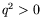.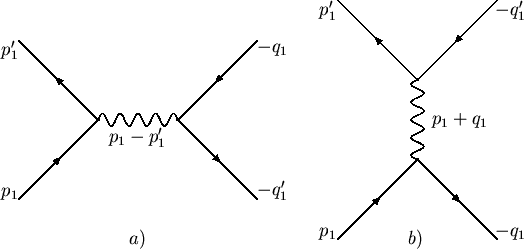We invoke the subsitution rule to obtain the cross-section from the Møller formula. With the substitutions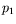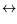(7.319)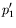(7.320)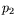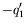(7.321)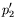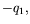(7.322)

and the change of sign in the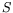-matrix we find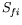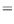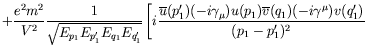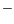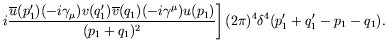(7.323)

The first term represents direct electron-positron scattering. The second term represents annihilation. Note the amplitude is antisymmetric this time.

In order to obtain the cross-section for electron-positron scattering in the centre-of-mass system, we apply the substitution rules and carry out the traces as for Møller scattering. Only the invariant matrix element changes. The four-momentum transfer in the denominator of the terms in the invariant matrix element become accoding to figure 7.13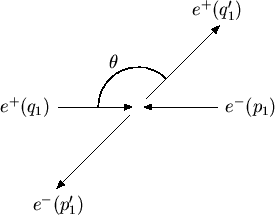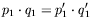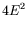(7.324)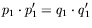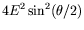(7.325)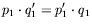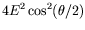(7.326)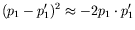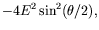(7.327)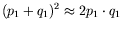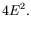(7.328)

The traces become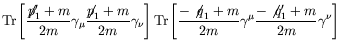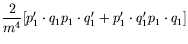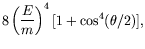(7.329)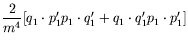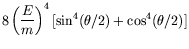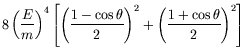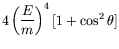(7.330)

and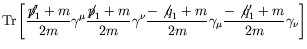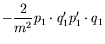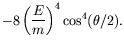(7.331)

Thus the invariant matrix element is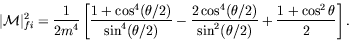(7.332)

In the extreme relativistic limit, the differential cross-section becomes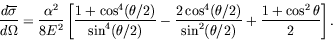(7.333)

For muon pair creation,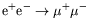, only the annhilation diagram can contribute because the initial and final states of particle-antiparticle pairs are of different types. In the ultra-relativistic limit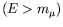the differential cross-section for muon pair creation thus becomes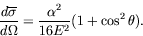(7.334)

Integration over the solid angle gives the total cross-section: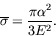(7.335)

Finally we note that quantum electrodynamics no longer gives a correct description of annihilation processes of the type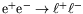(where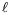referes to any lepton) if the available energy comes close to the mass of the neutral intermeidate vector boson Z (91 GeV). This particle can be produced on mass shell'' as a resonance (width 2.5 GeV) and then completely dominates over the contribution from the virtual photon.

The theory of electroweak interactions gives a unified description of both contributions. If the theoretically well understood contributions from the weak interaction is introduced, the predictions of quantum electrodynamics compare well with experimental data. From this we conclude that the electron is a point-like elementary particle. An extended composite object would be described by a momentum-dependent form-factor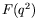. Experiments tell us that for the electron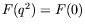up to momentum transfers of several hundress GeV. This implies that the electron is a point-like object down to a distance of at least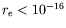cm. The same conculsion also holds for the heavy leptons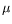and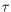.Next: Polarization in Electron Scattering Up: QED Processes Previous: Electron-Electron Scattering
Douglas M. Gingrich (gingrich@ ualberta.ca)
2004-03-18